# The Problem: Proper Prediction Intervals for Model Averaged Sets

## Prediction versus Fit Intervals

When we make predictions with fit statistical models, these models include two sources of error. The first, fit error, is error produced due to imprecise estimates of a coefficient. When we plot, say, a linear regression, this is what accounts for that beautiful bow-tie shape. The second source of error is residual error due to a model not being a perfect prediction of the data. When we use a model to predict new data, this later becomes very important for makign real-world predictions with realistic bounds of uncertainty.

## AIC and Optimizing for Prediction

When talking about prediction and multiple models, it’s all about AIC! AIC provides an estimate of out-of-sample (read: prediction) deviance for new sets of predictors. I highly recomment Richard McElreath’s chapter on this in his Statistical Rethinking - he really gives the cleanest explanation I’ve seen. Now, if you’re hip to current trends in AIC and multi-model inference, we’ve really hit a point where, if you have multiple models and a ‘best’ model isn’t super clear (and it rarely ever is), multi-model averaging of predicted values seems to be the way to go. Moreover, these model averaged values take into account not only parameter uncertainty, but also model uncertainty. Therefore, we get a wider confidence interval that should encompass a greater region of possible predicted values.

## Model Averaged Predictions and Confidence Intervals

The use of confidence intervals from model averaged sets does not, however, obviate the need to think about prediction uncertainty. Just because we are taking uncertainty in parameter estimates into account does not mean that predictions and error that accomodates model averaged coefficient error is going to provide a proper representation of error in new predictions. For example, let’s model `mpg` in the `mtcars` data set as a function of several predictors and compare it’s best model fit intervals, best model prediction intervals, and model averaged confidence intervals.

``library(tidyverse)``
``## ── Attaching packages ───────────────────────────────────────────── tidyverse 1.2.1 ──``
``````## ✔ ggplot2 2.2.1     ✔ purrr   0.2.4
## ✔ tibble  1.4.2     ✔ dplyr   0.7.4
## ✔ tidyr   0.8.0     ✔ stringr 1.3.0
## ✔ readr   1.1.1     ✔ forcats 0.3.0``````
``````## ── Conflicts ──────────────────────────────────────────────── tidyverse_conflicts() ──
``````library(modelr)
library(AICcmodavg)
library(ggplot2)
theme_set(theme_bw(base_size=14))

#let's make a few models

mod1 <- lm(mpg ~ cyl + hp + wt, data = mtcars)
mod2 <- lm(mpg ~ cyl, data = mtcars)
mod3 <- lm(mpg ~  hp, data = mtcars)
mod4 <- lm(mpg ~  wt, data = mtcars)
mod5 <- lm(mpg ~ cyl  + wt, data = mtcars)
mod6 <- lm(mpg ~ hp  + wt, data = mtcars)
mod7 <- lm(mpg ~ cyl  + hp, data = mtcars)
mod_null <- lm(mpg ~ 1, data = mtcars)

mod_list <- list(mod1, mod2, mod3, mod4, mod5,
mod6, mod7, mod_null)

#The AIC Table
aictab(mod_list)``````
``````##
## Model selection based on AICc:
##
##      K   AICc Delta_AICc AICcWt Cum.Wt      LL
## Mod5 4 157.49       0.00   0.38   0.38  -74.01
## Mod1 5 157.78       0.29   0.33   0.72  -72.74
## Mod6 4 158.13       0.64   0.28   1.00  -74.33
## Mod4 3 166.89       9.40   0.00   1.00  -80.01
## Mod2 3 170.16      12.67   0.00   1.00  -81.65
## Mod7 4 171.04      13.55   0.00   1.00  -80.78
## Mod3 3 182.10      24.60   0.00   1.00  -87.62
## Mod8 2 209.17      51.68   0.00   1.00 -102.38``````

So, we see that `mod5` is the best of the bunch, but there are several models that are just as good. Now, we can create a bunch of intervals as specified above based on best or model averaged sets. We’ll use `wt` as the predictor we’re interested in and average everything else.

``````#Data for new predictions based on
#current range of data looking only at
#effect of wt
new_data <- mtcars %>%
data_grid(wt = seq_range(wt, 100),
hp = mean(hp),
cyl = mean(cyl))

#best model fit intervals
best_fit <- new_data %>%
predict(mod5, newdata = ., interval = "confidence") %>%
cbind(new_data) %>%
mutate(type = "best_fit") %>%
rename(mpg = fit)

#best model prediction intervals
best_pred <- new_data %>%
predict(mod5, newdata = ., interval = "prediction") %>%
cbind(new_data) %>%
mutate(type = "best_predict") %>%
rename(mpg = fit)

#model averaged predictions
best_fit_modavg <- new_data %>%
modavgPred(mod_list, newdata = .) %>%
cbind(new_data) %>%
mutate(type = "best_fit_modavg") %>%
rename(mpg = mod.avg.pred,
lwr = lower.CL,
upr = upper.CL) %>%
select(type, wt, mpg, lwr, upr, uncond.se)``````
``````## Warning in modavgPred.AIClm(mod_list, newdata = .):
## Model names have been supplied automatically in the table``````
``````#plot!
ggplot(bind_rows(best_fit, best_pred, best_fit_modavg),
aes(x=wt, y = mpg, group = type,
color = type, ymin = lwr, ymax=upr,
fill = type)) +
geom_line() +
geom_ribbon(alpha=0.3, color=NA) ``````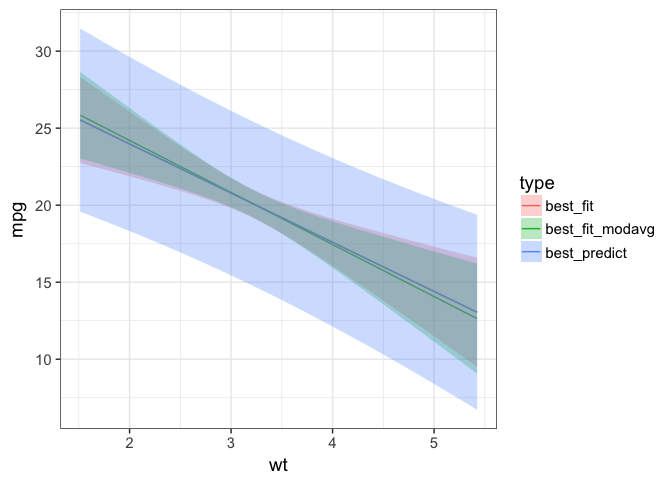So, looking here, we can see that the best fit prediction interval is far wider than anything else. The model averaged fit interval is slightly different than the best fit, but they’re actually broadly fairly similar, which is nice. Likely a function of `wt` being in all of the low AICc models.

But, the important thing here is that, while the model averaged set should give us the best estimate of uncertainty, it isn’t doing so with prediction intervals. The confidence intervals are about fit - and are far too narrow. What to do?

## A Bayesian Look at the Problem

In the Bayesian world, this isn’t a huge problem. When getting a distribution of predictions, we can use the residual variance and its distribution as just another parameter and make draws from the appropriate posterior. Leaving it out would just lead to a distribution of fit values, weighted by whatever IC we are using. For example, let’s look at the WAIC.

``````library(brms)

brm_mod1 <- brm(mpg ~ cyl + hp + wt, data = mtcars, chains=1)
brm_mod2 <- brm(mpg ~ cyl, data = mtcars, chains=1)
brm_mod3 <- brm(mpg ~  hp, data = mtcars, chains=1)
brm_mod4 <- brm(mpg ~  wt, data = mtcars, chains=1)
brm_mod5 <- brm(mpg ~ cyl  + wt, data = mtcars, chains=1)
brm_mod6 <- brm(mpg ~ hp  + wt, data = mtcars, chains=1)
brm_mod7 <- brm(mpg ~ cyl  + hp, data = mtcars, chains=1)
brm_mod_null <- brm(mpg ~ 1, data = mtcars, chains=1)``````
``````waic(brm_mod1, brm_mod2, brm_mod3, brm_mod4,
brm_mod5, brm_mod6, brm_mod7, brm_mod_null, compare=FALSE)``````
``````##                WAIC   SE
## brm_mod1     156.95 9.13
## brm_mod2     170.16 7.68
## brm_mod3     182.46 8.65
## brm_mod4     166.79 8.40
## brm_mod5     156.73 8.98
## brm_mod6     158.03 9.44
## brm_mod7     170.30 7.47
## brm_mod_null 208.78 7.84``````

We can see we have roughly the same model ordering - roughly. But, we can look at fit and prediction intervals from the posterior pretty easily. And compare prediction intervals between the best fit and th model average. Let’s start there.

``````brm_pred_avg <- pp_average(brm_mod1, brm_mod2, brm_mod3,
brm_mod4, brm_mod5, brm_mod6, brm_mod7,
brm_mod_null, weights = "WAIC", method = "predict",
newdata = new_data) %>%
cbind(new_data) %>%
mutate(type = "bayesian_modavg_predict") %>%
rename(mpg = Estimate, lwr = `2.5%ile`, upr = `97.5%ile`)

brm_pred_best <- predict(brm_mod5, newdata = new_data) %>%
cbind(new_data) %>%
mutate(type = "bayesian_best_predict") %>%
rename(mpg = Estimate, lwr = `2.5%ile`, upr = `97.5%ile`)

ggplot(rbind(brm_pred_best, brm_pred_avg),
aes(x=wt, y = mpg, group = type,
color = type, ymin = lwr, ymax=upr,
fill = type)) +
geom_line() +
geom_ribbon(alpha=0.3, color=NA) ``````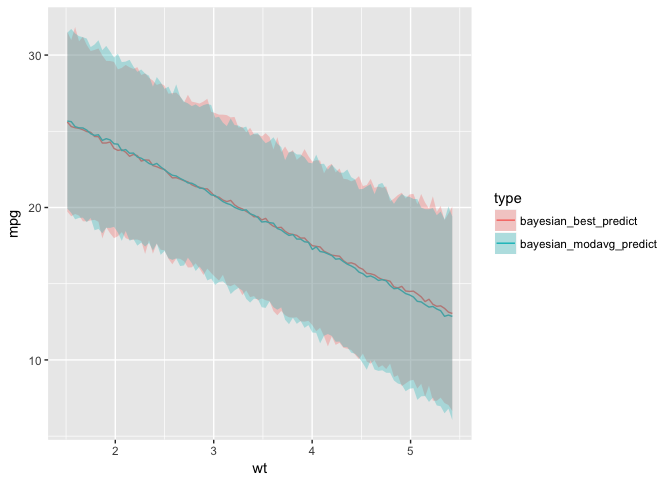They’re pretty similar. Again, not surprising given that `wt` is in all of the best models. We can also see it’s a pretty wide interval. If we want to compare it to the fit intervals, we can.

``````brm_fit_best <- fitted(brm_mod5, newdata = new_data) %>%
cbind(new_data) %>%
mutate(type = "bayesian_best_fit") %>%
rename(mpg = Estimate, lwr = `2.5%ile`, upr = `97.5%ile`)

brm_fit_avg <- pp_average(brm_mod1, brm_mod2, brm_mod3,
brm_mod4, brm_mod5, brm_mod6, brm_mod7,
brm_mod_null, weights = "WAIC", method = "fitted",
newdata = new_data)%>%
cbind(new_data) %>%
mutate(type = "bayesian_modavg_fit") %>%
rename(mpg = Estimate, lwr = `2.5%ile`, upr = `97.5%ile`)

ggplot(rbind(brm_fit_best, brm_pred_best, brm_fit_avg, brm_pred_avg),
aes(x=wt, y = mpg, group = type,
color = type, ymin = lwr, ymax=upr,
fill = type)) +
geom_line() +
geom_ribbon(alpha=0.3, color=NA)  + facet_wrap(~type)``````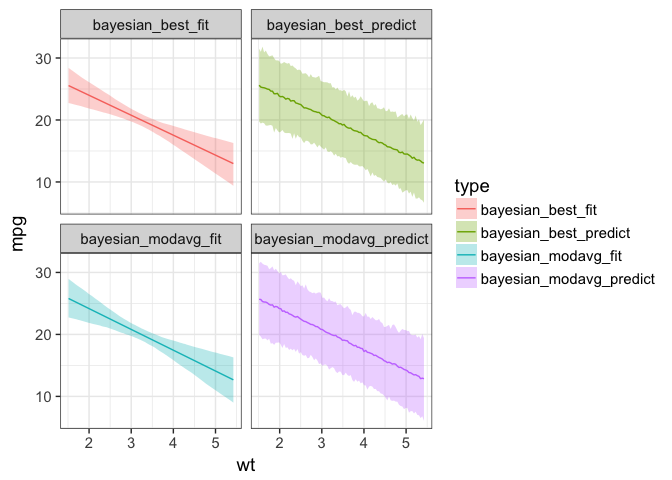OK, so, this works with posteriors - we should be able to do this with likelihood-based fits.

## Prediction Intervals and Model Averaging

There are a few possible solutions to doing this in a likelihood-least squares world. One could be getting a model averaged residual SD and working from there, but, that’s not easily generalized to other distributions. Perhaps the simples and likely correct is to just get model averaged confidence intervals of a prediction and average those! It works pretty well - one has to calculate weights, then get prediction intervals from each model, and then get weighted intervals. We can do this with some `purrr` magic pretty easily.

``aicc_weights <- aictab(mod_list, sort=FALSE)\$AICcWt``
``````## Warning in aictab.AIClm(mod_list, sort = FALSE):
## Model names have been supplied automatically in the table``````
``````#get predictions from each model
#multiply them by weights
#then sum eveything
pred_intervals <- map(mod_list, predict, newdata = new_data, interval="prediction") %>%
map(as_tibble) %>%
map(~select(., -fit)) %>%
map2(aicc_weights, `*`) %>%
reduce(`+`)

best_pred_modavg_weightpred <- bind_cols(best_fit_modavg %>% select(wt, mpg), pred_intervals) %>%
mutate(type = "best_pred_modavg_weightpred")``````

Voila! Now let’s plot it as we did in the Bayesian case to see how it all looks.

First, prediction interval to prediction interval

``````ggplot(bind_rows(best_pred, best_pred_modavg_weightpred),
aes(x=wt, y = mpg, group = type,
color = type, ymin = lwr, ymax=upr,
fill = type)) +
geom_line() +
geom_ribbon(alpha=0.3, color=NA)``````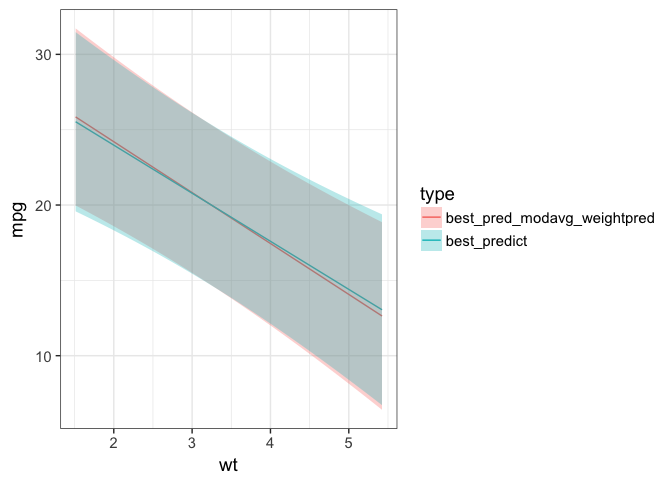Nice overlap! In fact, let’s see that with the Baysian intervals

``````ggplot(bind_rows(best_pred, best_pred_modavg_weightpred, brm_pred_best, brm_pred_avg),
aes(x=wt, y = mpg, group = type,
color = type, ymin = lwr, ymax=upr,
fill = type)) +
geom_line() +
geom_ribbon(alpha=0.3, color=NA)``````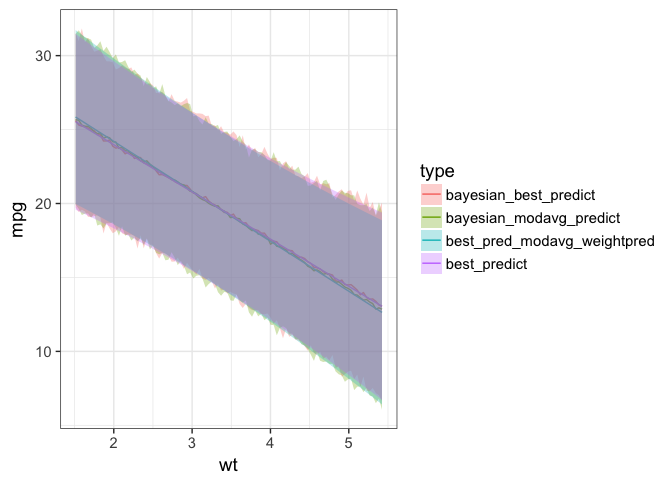I love how well everything in this plot lines up.

For our final look, let’s compare fit and prediction intervals here.

``````ggplot(bind_rows(best_pred, best_pred_modavg_weightpred, best_fit, best_fit_modavg),
aes(x=wt, y = mpg, group = type,
color = type, ymin = lwr, ymax=upr,
fill = type)) +
geom_line() +
geom_ribbon(alpha=0.3, color=NA) +
facet_wrap(~type)``````